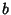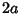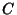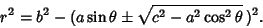Watt's CurveA curve named after James Watt (1736-1819), the Scottish engineer who developed the steam engine (MacTutor Archive). The curve is produced by a Linkage of rods connecting two wheels of equal diameter. Let the two wheels have Radiusand let their centers be located a distanceapart. Further suppose that a rod of lengthis fixed at each end to the Circumference of the two wheels. Letbe the Midpoint of the rod. Then Watt's curveis the Locus of.

The Polar equation of Watt's curve isIf, thenis a Circle of Radiuswith a figure of eight inside it.

References

Lockwood, E. H. A Book of Curves. Cambridge, England: Cambridge University Press, p. 162, 1967.

MacTutor History of Mathematics Archive. Watt's Curve.'' http://www-groups.dcs.st-and.ac.uk/~history/Curves/Watts.html.